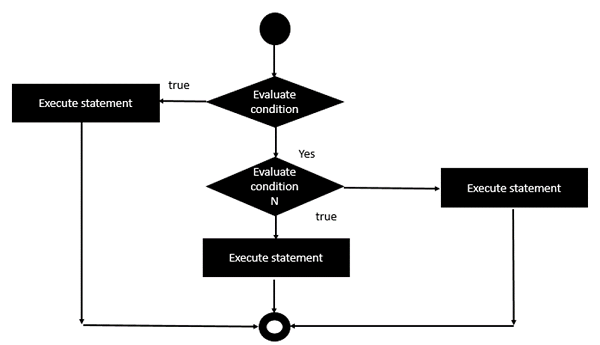# Erlang - Nested if Statements

Sometimes, there is a requirement to have multiple if statements embedded inside of each other, as is possible in other programming languages. In Erlang also this is possible.

The following image is a diagram representation of the Nested if statement.An example of this is shown in the following program −

## Example

```-module(helloworld).
-export([start/0]).

start() ->
A = 4,
B = 6,
if
A < B ->
if
A > 5 ->
io:fwrite("A is greater than 5");
true ->
io:fwrite("A is less than 5")
end;
true ->
io:fwrite("A is greater than B")
end.
```

In the above program the following point should be noted −

• When the first if condition is evaluated to true, then it starts the evaluation of the second if condition.

The output of the above code will be −

## Output

```A is less than 5
```
erlang_decision_making.htm